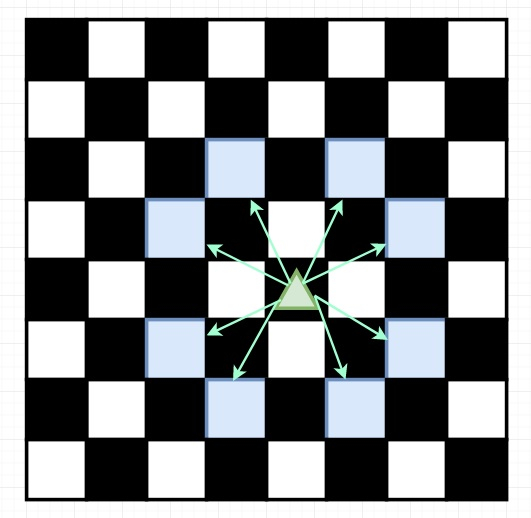# Minimum Knight Moves in C++

Suppose we have an infinite chessboard with coordinates from -infinity to +infinity, and we have a knight at square [0, 0]. A knight has 8 possible moves it can make, as shown below. Each move is two squares in a cardinal direction, then one square in an orthogonal direction.We have to find the minimum number of steps needed to move the knight to the square [x, y]. It is guaranteed the answer exists.

So if the input is like x = 5 and y = 5, then the output will be 4. This will be like [0,0] → [2,1] → [4,2] → [3,4] → [5,5]

To solve this, we will follow these steps −

• Define a map m

• define a method called solve(), this will take x and y

• if x + y = 0, then return 0, if x + y = 2, then return 2

• make a pair temp using (x, y)

• if m has the pair temp, then return m[temp]

• return m[temp] := min of solve(|x - 1|, |y - 2|)), [solve(|x - 2|, |y - 1|)) + 1]

• From the main method, call solve(|x|, |y|), and return its value

## Example (C++)

Let us see the following implementation to get a better understanding −

Live Demo

#include <bits/stdc++.h>
using namespace std;
class Solution {
public:
map < pair <int, int>, int > dp;
int solve(int x, int y){
if(x + y == 0) return 0;
if (x + y == 2) return 2;
pair <int, int> temp({x, y});
if(dp.count(temp)) return dp[temp];
return dp[temp] = min(solve(abs(x - 1), abs(y - 2)), solve(abs(x - 2), abs(y - 1))) + 1;
}
int minKnightMoves(int x, int y) {
return solve(abs(x), abs(y));
}
};
main(){
Solution ob;
cout << (ob.minKnightMoves(5, 5));
}

## Input

5
5

## Output

4